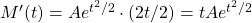# 5.7: Initial Value Problems

$$\newcommand{\vecs}{\overset { \rightharpoonup} {\mathbf{#1}} }$$ $$\newcommand{\vecd}{\overset{-\!-\!\rightharpoonup}{\vphantom{a}\smash {#1}}}$$$$\newcommand{\id}{\mathrm{id}}$$ $$\newcommand{\Span}{\mathrm{span}}$$ $$\newcommand{\kernel}{\mathrm{null}\,}$$ $$\newcommand{\range}{\mathrm{range}\,}$$ $$\newcommand{\RealPart}{\mathrm{Re}}$$ $$\newcommand{\ImaginaryPart}{\mathrm{Im}}$$ $$\newcommand{\Argument}{\mathrm{Arg}}$$ $$\newcommand{\norm}{\| #1 \|}$$ $$\newcommand{\inner}{\langle #1, #2 \rangle}$$ $$\newcommand{\Span}{\mathrm{span}}$$ $$\newcommand{\id}{\mathrm{id}}$$ $$\newcommand{\Span}{\mathrm{span}}$$ $$\newcommand{\kernel}{\mathrm{null}\,}$$ $$\newcommand{\range}{\mathrm{range}\,}$$ $$\newcommand{\RealPart}{\mathrm{Re}}$$ $$\newcommand{\ImaginaryPart}{\mathrm{Im}}$$ $$\newcommand{\Argument}{\mathrm{Arg}}$$ $$\newcommand{\norm}{\| #1 \|}$$ $$\newcommand{\inner}{\langle #1, #2 \rangle}$$ $$\newcommand{\Span}{\mathrm{span}}$$$$\newcommand{\AA}{\unicode[.8,0]{x212B}}$$

Often a differential equation has many solutions. Consider the population equation

$$P'(t) = 0.03 P(t)$$

We saw in the last section that $$P(t) = e^{0.03 t}$$ solves this differential equation. However, $$73e^{0.03t}$$ also solves this differential equation:

\begin{align*} P'(t) & = 73 e^{0.03t} \cdot 0.03 \\ & = 0.03 \cdot 73 e^{0.03t} \\ & = 0.03 P(t) \end{align*}

So $$e^{0.03 t}$$ and $$73 e^{0.03t}$$ both solve the differential equation $$P'(t) = 0.03 P(t)$$. In fact, any function of the form $$P(t) = A e^{0.03t}$$ solves this differential equation. $$A e^{0.03t}$$ is called the general solution, and $$A$$ is called a free parameter, since it can be anything that we like. However, sometimes there are certain conditions called initial conditions which specify what the free parameters must be (in which case it wouldn’t be very free!). A differential equation with given initial conditions is called an initial value problem. I won’t ask you to solve the differential equation fully in this book, but solving for the free parameters is very doable.

## Initial Value Problem

Solve $$P'(t) = 0.03 P(t)$$ where $$P(0) = 2050$$. (Hint: The general solution is $$P(t) = Ae^{0.03t}$$).

In this case, we just need to specify what $$A$$, since it is the only free parameter. We see that $$P(t) = A e^{0.03 t}$$ and $$P(0) = 2050$$. Therefore, $$A e^{0.03 \cdot 0} = 2050$$. Anything to the zero is $$1$$, hence we see $$A (1) = 2050$$, so $$A = 2050$$. So the final answer is $$\boxed{P(t) = 2050 e^{0.03t}}$$.

## Another Initial Value Problem

Verify $$M'(t) = t M(t)$$. Then solve for the free parameter if $$M(0) = -4$$.

We see that $$M'(t)$$ using the chain rule:. But notice this exactly fits the differential equation:Thus we have verified the solution to the differential equation. To find the free parameter $$A$$, we use $$M(0) = -4$$, and see that $$A (1) = -4$$, and so $$A = \boxed{-4}$$. This completes the verification and solving for the free parameter.

This page titled 5.7: Initial Value Problems is shared under a CC BY 4.0 license and was authored, remixed, and/or curated by Tyler Seacrest via source content that was edited to the style and standards of the LibreTexts platform; a detailed edit history is available upon request.